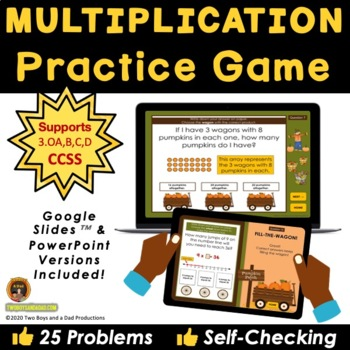# Multiplication Practice Game on Google Slides3rd - 5th
Subjects
Standards
Resource Type
Formats Included
• Zip
•Google Apps™
Pages
45 slides + PDFsThe Teacher-Author indicated this resource includes assets from Google Workspace (e.g. docs, slides, etc.).

### Description

Third graders can practice multiplication facts with this self-checking game played on Google Slides™. This Pumpkin Patch theme multiplication game has 25 problems that include using equal groups, arrays, and number lines. This multiplication facts game is self-paced and adaptable for in-person or distance learning situations.

HOW IT WORKS

Assign the Google Slides™ game in Google Classroom™ or Microsoft Teams (PowerPoint version included). A one-page convenient PDF with a clickable link takes the student directly to the game in the PRESENT mode.

• Then the student plays the game on any device that supports Google Slides™ or PowerPoint. Each question is self-checking. Students are provided with immediate feedback if the response is correct or incorrect. If incorrect, students get another chance.

• For accountability, students can write their answers on any piece of paper or use the included PDF Answer Sheet that is printable and is also a fillable PDF form.

• Once the student has completed all 25 problems, the student will see a congratulatory slide for finishing the game. The game can be replayed over and over again.

• Most problems have a Depth of Knowledge Level 1, with some at Level 2.

WHAT’S INCLUDED

• Google Slides™ version of the game
• PowerPoint version of the game
• 5 problems using equal groups
• 5 problems using arrays
• 6 problems using number lines
• 5 problems using the Properties of Multiplication
• 4 two-step word problems involving multiplication, addition, and subtraction
• Google Slides™ Student Instruction Page - a one-page PDF that has a clickable link to take the student directly to the game in the PRESENT mode
• Teachers instructions for assigning the game
• List of types of problems

Related Products

*******************************************************************

Comments, suggestions, feedback, and questions always welcomed!

*******************************************************************

♦ Be sure to FOLLOW ME for updates and new product announcements!

Do you need some fresh ideas and tips? Then visit My BLOG

♦ Each time you give feedback, TPT gives you feedback credits that you use to lower the cost of your future purchases. I value your feedback greatly!

♦ If you have any questions or concerns, go ahead and contact me through the Q & A and I'll get back to you ASAP!

*******************************************************************

Total Pages
45 slides + PDFs
Included
Teaching Duration
N/A
Report this Resource to TpT
Reported resources will be reviewed by our team. Report this resource to let us know if this resource violates TpT’s content guidelines.

### Standards

to see state-specific standards (only available in the US).
Solve two-step word problems using the four operations. Represent these problems using equations with a letter standing for the unknown quantity. Assess the reasonableness of answers using mental computation and estimation strategies including rounding.
Fluently multiply and divide within 100, using strategies such as the relationship between multiplication and division (e.g., knowing that 8 × 5 = 40, one knows 40 ÷ 5 = 8) or properties of operations. By the end of Grade 3, know from memory all products of two one-digit numbers.
Apply properties of operations as strategies to multiply and divide. Examples: If 6 × 4 = 24 is known, then 4 × 6 = 24 is also known. (Commutative property of multiplication.) 3 × 5 × 2 can be found by 3 × 5 = 15, then 15 × 2 = 30, or by 5 × 2 = 10, then 3 × 10 = 30. (Associative property of multiplication.) Knowing that 8 × 5 = 40 and 8 × 2 = 16, one can find 8 × 7 as 8 × (5 + 2) = (8 × 5) + (8 × 2) = 40 + 16 = 56. (Distributive property.)
Determine the unknown whole number in a multiplication or division equation relating three whole numbers. For example, determine the unknown number that makes the equation true in each of the equations 8 × ? = 48, 5 = __ ÷ 3, 6 × 6 = ?.
Use multiplication and division within 100 to solve word problems in situations involving equal groups, arrays, and measurement quantities, e.g., by using drawings and equations with a symbol for the unknown number to represent the problem.